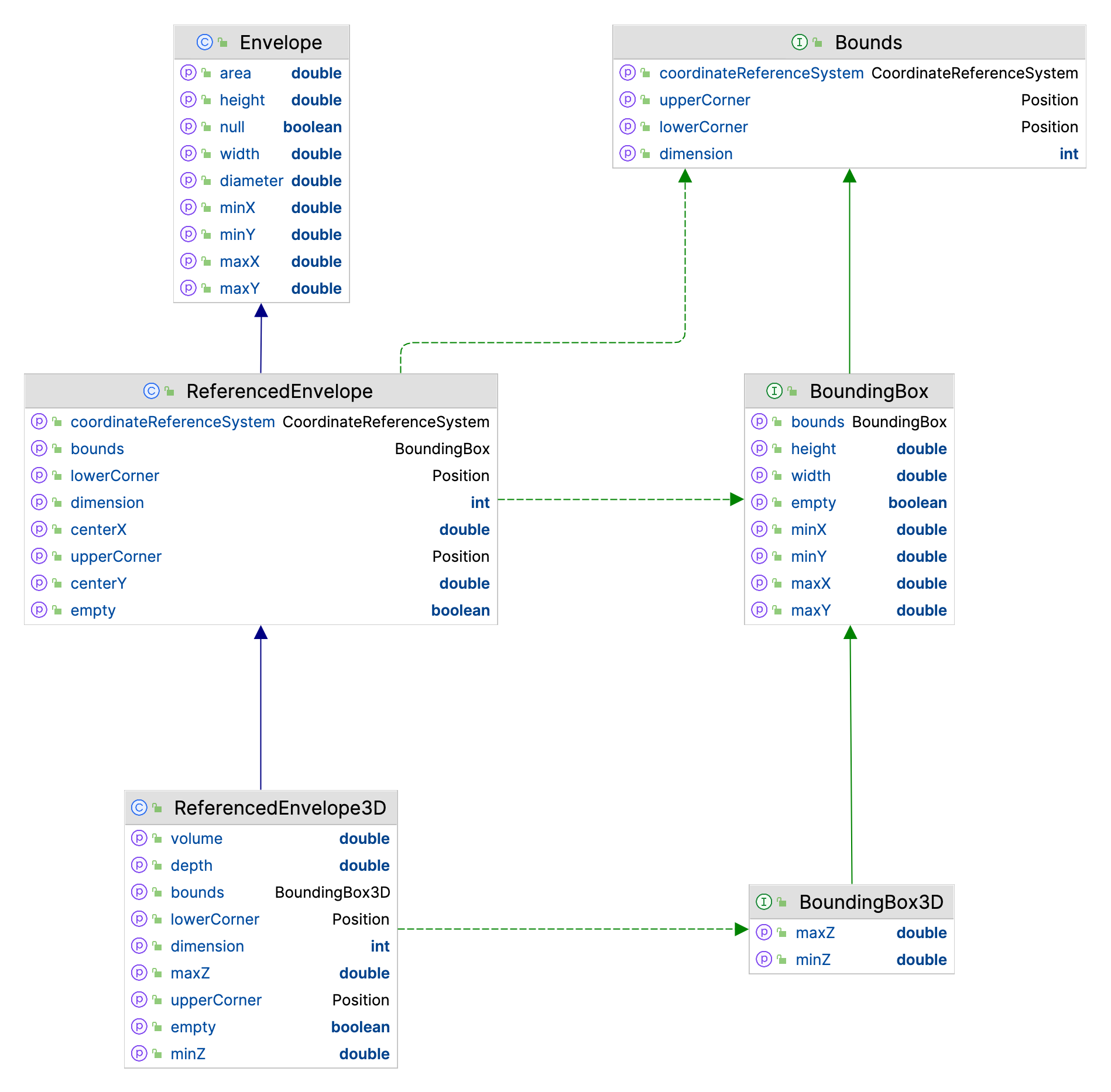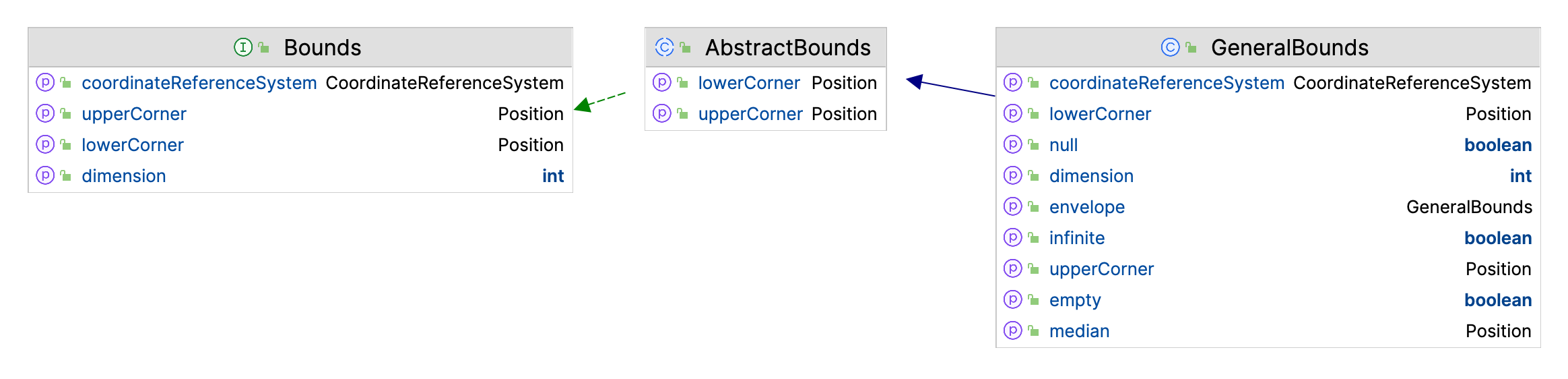# Bounds¶

Bounds are used to represent the extent of a geometry, and are used very frequently as a way to quickly check if a geometry is in the area you are interested in.GeoTools, through virtue of reusing code, has seveal “Bounds” implementations to contend with:

• `gt-api` module `Bounds` and `Bounds3D` representing a spatial extent, complete with `CoordinateReferenceSystem`.

The Coverage API in `gt-api` package `org.geotools.api.coverage` makes use of `Bounds` and `Bounds3D` extensively.

• `gt-api` module `BoundingBox` and `BoundingBox3D` represent a spatial extent, assuming `CoordinateReferenceSystem` defines axis in `X/Y` order.

• `gt-referencing` implements `AbstractBounds` and `GeneralBounds`, to the area of validity for a coordinate reference system.

• `gt-main` defines `ReferencedEnvelope` which is adds a `CoordinateReferenceSystem` to a JTS Topology Suite `Envelope`. The class `ReferencedEnvelope3D` supports a third dimension on top of the regular two dimensions.

DataStore API `gt-main` packages `org.geotools.api.data` makes use of `ReferencedEnvelope` and `ReferencedEnvelope3D` extensively (integrating well with use of JTS `Geometry`).

You will find other “Rectangles” around as you make use of GeoTools in a real world application.

• Java `Rectangles`

Java `Rectangles` record x,y,w,h:

• `Rectangle2D`

• `Rectangle2D.Double` rectangle working with doubles

• `Rectangle2D.Float` rectangle working with floats

• `GeneralBounds` we have a spatial specific version of `Rectangle2D` that implements ISO Geometry Envelope

• `Rectangle` the original `java.awt` rectangle for working on the screen, measured in integer pixels.

## ReferencedEnvelope and ReferencedEnvelope3D¶

GeoTools `ReferencedEnvelope` extends JTS `Envelope` to implement the `gt-api` module `Bounds` interface, and subclass `ReferencedEnvelope3D` implementing `Bounds3D`.Use of `ReferencedEnvelope` is the most common representation of a bounds in GeoTools:

```ReferencedEnvelope envelope =
new ReferencedEnvelope(0, 10, 0, 20, DefaultGeographicCRS.WGS84);

double xMin = envelope.getMinX();
double yMin = envelope.getMinY();

double xMax = envelope.getMaxX();
double yMax = envelope.getMaxY();

double width = envelope.getWidth();
double height = envelope.getHeight();

double xCenter = envelope.getMedian(0);
double yCenter = envelope.getMedian(1);

CoordinateReferenceSystem crs = envelope.getCoordinateReferenceSystem();
int dimension = envelope.getDimension();

Position lower = envelope.getLowerCorner();
Position upper = envelope.getUpperCorner();

// expand to include 15, 30
envelope.include(15, 30);

envelope.isEmpty(); // check if storing width and height are 0

envelope.isNull(); // check if "null" (not storing anything)
envelope.setToNull();
```

## Bounds and GeneralBounds¶

The `gt-api` module records `Bounds` as an extent measured along each axis mentioned by the `CoordinateReferenceSystem` object. You can use this approach to record a simple rectangle in space, a height range, and a range in time as needed.

The `GeneralBounds` implementation records spans in multiple dimensions (think depth, height or time).Since``Bounds`` is an interface we will work with GeneralBounds in this example:

```CoordinateReferenceSystem wsg84 = CRS.decode("EPSG:4326");

GeneralPosition lowerPosition = new GeneralPosition(0.0, 0.0);
lowerPosition.setCoordinateReferenceSystem(wsg84);

GeneralPosition upperPosition = new GeneralPosition(10.0, 20.0);
upperPosition.setCoordinateReferenceSystem(wsg84);

Bounds bounds = new GeneralBounds(lowerPosition, upperPosition);

double xMin = bounds.getMinimum(0);
double yMin = bounds.getMinimum(1);

double xMax = bounds.getMaximum(0);
double yMax = bounds.getMaximum(1);

double width = bounds.getSpan(0);
double height = bounds.getSpan(1);

double xCenter = bounds.getMedian(0);
double yCenter = bounds.getMedian(1);

CoordinateReferenceSystem crs = bounds.getCoordinateReferenceSystem();

Position lower = bounds.getLowerCorner();
Position upper = bounds.getUpperCorner();

// expand to include 15, 30
lower.setOrdinate(0, Math.min(lower.getOrdinate(0), 15));
lower.setOrdinate(1, Math.min(lower.getOrdinate(1), 30));
upper.setOrdinate(0, Math.max(upper.getOrdinate(0), 15));
upper.setOrdinate(1, Math.max(upper.getOrdinate(1), 30));
```

Even in a simple example we should consult the CRS to define what each axis is measuring (the variables showing xMin and yMin hold unexpected values if the `CoordinateReferencSystem` defines Axis `0` as `NORTHING`)

## BoundingBox and BoundingBox3D¶

If you are confident that you are working with data in X/Y order you can directly make use of `BoundingBox` box. `BoundingBox` is an extension of `Envelope` for working with 2D data, and it has been made method compatible with JTS `Envelope` where possible.

Since `BoundingBox` is just an interface, so we will use `ReferencedEnvelope` for this example:

```CoordinateReferenceSystem wsg84 = CRS.decode("EPSG:4326");
org.geotools.api.geometry.BoundingBox bbox = new ReferencedEnvelope(0, 10, 0, 20, wsg84);

double xMin = bbox.getMinX();
double yMin = bbox.getMinY();

double xMax = bbox.getMaxX();
double yMax = bbox.getMaxY();

double width = bbox.getWidth();
double height = bbox.getHeight();

double xCenter = bbox.getMedian(0);
double yCenter = bbox.getMedian(1);

CoordinateReferenceSystem crs = bbox.getCoordinateReferenceSystem();

Position lower = bbox.getLowerCorner();
Position upper = bbox.getUpperCorner();

// expand to include 15, 30
bbox.include(15, 30);
```

## JTS Envelope¶

The JTS Topology Suite has the concept of an `Envelope` recorded in `x1,x2, y1,y2` order.

You can see that the use of JTS `Envelope` has the same “assumptions” as the use of `BoundingBox` above.:

```org.locationtech.jts.geom.Envelope envelope = new Envelope(0, 10, 0, 20);
double xMin = envelope.getMinX();
double yMin = envelope.getMinY();

double xMax = envelope.getMaxX();
double yMax = envelope.getMaxY();

double width = envelope.getWidth(); // assuming axis 0 is easting
double height = envelope.getHeight(); // assuming axis 1 is nothing

// Expand an existing envelope
Envelope bbox = new Envelope();
envelope.expandToInclude(bbox);

// Use
envelope.covers(5, 10); // inside or on edge!
envelope.contains(5, 10); // inside only

// Null
envelope.isNull(); // check if "null" (not storing anything)
envelope.setToNull();
```

Transform an `Envelope` using the JTS `Utility` class:

```CoordinateReferenceSystem sourceCRS = CRS.decode("EPSG:4326");
CoordinateReferenceSystem targetCRS = CRS.decode("EPSG:23032");

Envelope envelope = new Envelope(0, 10, 0, 20);

MathTransform transform = CRS.findMathTransform(sourceCRS, targetCRS);

Envelope quick = JTS.transform(envelope, transform);

// Sample 10 points around the envelope
Envelope better = JTS.transform(envelope, null, transform, 10);
```